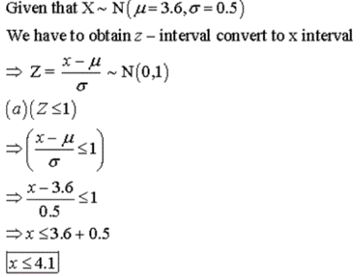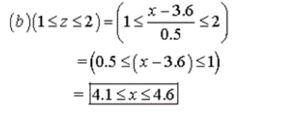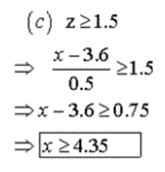# Convert each of the following z intervals to raw score x intervals. (a) z less than or equal to...

## Question:

Let {eq}x {/eq} be a random variable that represents the length of time it takes a student to complete Dr. Gill's chemistry lab project. From long experience, it is known that {eq}x {/eq} has a normal distribution with {eq}\mu = 3.6 {/eq} hours and {eq}\sigma = 0.5 {/eq} hour.

Convert each of the following {eq}z {/eq} intervals to raw score {eq}x {/eq} intervals.

(a) {eq}z \leq -1 {/eq}

(b) {eq}1 \leq z \leq 2 {/eq}

(c) {eq}z \geq 1.5 {/eq}

## Z-Statistics:

In statistical methods, the term Z-statistics is a kind of statistical test in which the two population means are not the same, the variance is known, and the sample size is greater than 30.